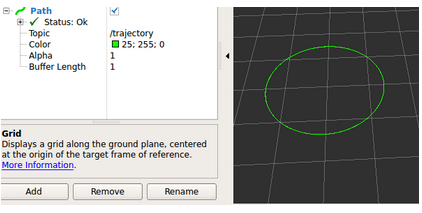•5星
2KB weixin_45479707 2021-06-30 14:17:26
•5星
10.46MB xipengbozai 2021-03-22 00:43:45
•5星
100KB lijj1985611 2021-04-01 16:10:28
•5星
21.17MB qc_id_01 2021-07-29 12:05:14
•5星
133KB weixin_43328149 2021-06-20 16:55:21
•5星
43KB qq_34093397 2021-01-04 10:45:38
•5星
63KB weixin_42696333 2021-09-10 15:29:31
•5星
12.63MB qq_41113628 2021-03-31 21:33:42
•5星
48.61MB goodxianping 2021-09-23 09:41:55
•5星
81.88MB u010611301 2020-11-23 14:22:28
• 9KB qq_36697127 2017-08-15 08:12:22
• 前言总结梳理常见曲线的参数方程；其中抛物线和双曲线的参数方程不要求掌握；参数方程一般地，在平面直角坐标系中，如果曲线$$C$$上任意一点$$P$$的坐标$$x$$、$$y$$都是某个变数$$t$$的函数：$\left\{\begin{array... 前言 总结梳理常见曲线的参数方程；其中抛物线和双曲线的参数方程不要求掌握； 参数方程 一般地，在平面直角坐标系中，如果曲线$$C$$上任意一点$$P$$的坐标$$x$$、$$y$$都是某个变数$$t$$的函数： \[\left\{\begin{array}{l}{x=f(t)}\\{y=g(t)}\end{array}\right.$

并且对于$$t$$的每一个允许的取值，由方程组确定的点$$(x, y)$$都在这条曲线$$C$$上，那么这个方程就叫做曲线的参数方程，联系变数$$x$$、$$y$$的变数$$t$$叫做参变数，简称参数。相对而言，直接给出点坐标间关系的方程叫普通方程。

例如在运动学，参数通常是“时间”，而方程的结果是速度、位置等。用参数方程描述运动规律时，常常比用普通方程更为直接简便。对于解决求最大射程、最大高度、飞行时间或轨迹等一系列问题都比较理想。有些重要但较复杂的曲线[例如摆线]，建立它们的普通方程比较困难，甚至不可能，有了参数方程，就可以很容易表达。

直线参数方程

直线的参数方程的形式不唯一，当选定的参数不一样时，参数方程的形式也就不一样了。

[方式1]：已知直线所过的定点$$(x_0，y_0)$$和倾斜角$$\theta$$，则以定点到动点$$(x，y)$$的有向线段的位移为参数，可知

直线的参数方程为$$\left\{\begin{array}{l}{x=x_0+cos\theta\cdot t}\\{y=y_0+sin\theta\cdot t}\end{array}\right.$$

[方式2]：以定比分点为参数

[方式3]：以曲线$$M$$上的点与点$$O$$连线的斜率为参数，

以坐标原点$$O$$为极点，$$x$$轴的正半轴为极轴建立极坐标系，已知曲线$$C$$的极坐标方程为$$\rho=4cos\theta$$，曲线$$M$$的直角坐标方程为$$x-2y+2=0(x>0)$$，以曲线$$M$$上的点与点$$O$$连线的斜率为参数，写出曲线$$M$$的参数方程；

分析：由$$\left\{\begin{array}{l}{x-2y+2=0(x>0)①}\\{y=kx②}\end{array}\right.$$

解方程，消去$$y$$，解得$$x=\cfrac{2}{2k-1}$$，代入②得到，$$y=\cfrac{2k}{2k-1}$$，由$$x=\cfrac{2}{2k-1}>0$$，得到$$k>\cfrac{1}{2}$$

故曲线$$M$$的参数方程为$$\left\{\begin{array}{l}{x=\cfrac{2}{2k-1}}\\{y=\cfrac{2k}{2k-1}}\end{array}\right.$$ ($$k$$为参数，$$k>\cfrac{1}{2}$$)

圆参数方程

圆$$(x-1)^2+(y-2)^2=4$$的参数方程为$$\left\{\begin{array}{l}{x=1+2cos\theta}\\{y=2+2sin\theta}\end{array}\right.\quad$$ ($$\theta$$为参数)

椭圆参数方程

椭圆$$\cfrac{x^2}{4^2}+\cfrac{y^2}{3^2}=1$$的的参数方程为$$\left\{\begin{array}{l}{x=4cos\theta}\\{y=3sin\theta}\end{array}\right.\quad$$ ($$\theta$$为参数)

抛物线参数方程

抛物线$$y^2=4x$$的参数方程为$$\left\{\begin{array}{l}{x=4t^2}\\{y=4t}\end{array}\right.\quad$$ ($$t$$为参数)

双曲线参数方程

双曲线$$\cfrac{x^2}{4^2}-\cfrac{y^2}{3^2}=1$$的的参数方程为$$\left\{\begin{array}{l}{x=4sec\theta}\\{y=3tan\theta}\end{array}\right.\quad$$ ($$\theta$$为参数)

展开全文weixin_39639381 2021-01-17 15:59:12
• 2.24MB yhsbzl 2021-09-09 05:12:36
• 三、常见曲线的参数方程第二章 轨迹与方程§1 平面曲线的方程§3　母线平行于坐标轴的柱面方程§4 空间曲线的方程§2　曲面的方程§1 平面曲线的方程一、曲线的方程二、曲线的参数方程三、常见曲线的参数方程一、...

三、常见曲线的参数方程

第二章 轨迹与方程

§1 平面曲线的方程

§3　母线平行于坐标轴的柱面方程

§4 空间曲线的方程

§2　曲面的方程

§1 平面曲线的方程

一、曲线的方程

二、曲线的参数方程

三、常见曲线的参数方程

一、曲线的方程

定义1

当平面上取定了坐标系之后，如果一个方程与一条曲线之

间有着关系：

①满足方程的 必是曲线上某一点的坐标；

②曲线上任何一点的坐标 满足这个方程，

那么这个方程就叫做这条曲线的方程，这条曲线叫做这个

方程的图形。

概括而言，曲线上的点与方程之间有着一一对应的关系

例1 求圆心在原点，半径为R 的圆的方程

例2 已知两点 和 ，求满足条件

的动点M 的轨迹方程

二、曲线参数的方程

定义2

若取 的一切可能取值

①由 表示的向径 的终点总在一条曲线上

②在这条曲线上的任意点，总对应着以它为终点的向径，而这向径可由

的某一值 通过 完全决定

那么就把 叫做曲线的向量式参数方程，

其中 为参数。

其坐标式参数方程为

例3 一个圆在一直线上无滑动地滚动，求圆周上一定点的轨迹

该定点的轨迹为旋轮线或摆线(cycloid)

三、常见曲线的参数方程

(1) 一个半径为r 的小圆在半径为R 的大圆内无滑动地滚动，小圆周上一

定点P 的运动轨迹称为内摆线(hypocycloid)

例4 已知大圆半径为a ，小圆半径为b，设大圆不动，而小圆在大圆内无滑动地滚动，求动圆周上某一定点P 的轨迹方程

(a＝4b)四尖点星形线(astroid)

圆的内摆线

(2)一个半径为r的小圆在半径为R的大圆外无滑动地滚动，小圆周上一个定点P的运动轨迹称为外摆线(epicycloid)

其参数方程为

特别当R＝r时可以得到心脏线(cardioid)

其参数方程为

(3)把线绕在一个固定的圆周上，将线头拉紧后向反方向旋转，以把线从圆周上解放出来，使放出来的部分成为圆的切线，则线头的轨迹所形成的曲线叫做圆的渐伸线或切展线(involute)

其坐标式参数方程为

(4)椭圆的参数方程

设椭圆的方程为

第一种参数方程以角度 为参数：

第二种参数方程以斜率 为参数：

作业

P77 2 , 3

§2 曲面的方程

一、曲面的方程

二、曲面的参数方程

三、球坐标系与柱坐标系

一、曲面的方程

例1 求联结两点A(1,2,3)和B(2,-1,4)的线段的垂直平分面的方程.

例2 求两坐标面xOz 和yOz 所成二面角的平分面方程.

例3 求坐标平面yOz 的方程.

例4 一平面平行于坐标平面xOz，且在y 轴的正向一侧与平面xOz 相隔距离为k ，求它的方程.

例5 设球面的中心是点C(a,b,c)，而且半径等于r ，求它的方程.

求曲线方程一般需要下面的5个步骤：

1)选取适当的坐标系(如题中已给定，这一步可省)；

2)在曲线上任取一点，也就是轨迹上的流动点；

3)根据曲线上的点所满足的几何条件写出等式；

4)用点的坐标x,y,z的关系来表示这个等式，并化简得方程；

5)证明所得的方程就是曲线的方程，也就是证明它符合定义.

二、曲面的参数方程

例6 求球心在原点，半径为r 的球面的参数方程.

例7 求以z 轴为对称轴，半径为R 的圆柱面的参数方程.

结论 求空间曲面或曲线的参数方程时，经常是作向径 的坐标折线，将分解 为平行于坐标轴的三个向量之和，这样便于找出 x,y,z 与参数之间的函数关系.

注意 空间曲面的参数方程的表达式不是惟一的.

一般按下列三个步骤进行：

三、球坐标系与柱坐标系

1.球坐标系

2.柱坐标系

作业

P87～88

2(4) ， 3(3)，4(3)

§3 母线平行于坐标轴

的柱面方程

抛物柱面

平面

抛物柱面方程：

平面方程：

从柱面方程看柱面的特征：

(其他类推)

实 例

椭圆柱面，

双曲柱面 ，

抛物柱面，

母线//

展开全文weixin_39798943 2021-01-17 15:59:13
• 运动控制领域两种常见的速度曲线，T型和S型曲线 下面介绍T型曲线，T型曲线的速度曲线是一个T型。 在运动控制应用中，在位置控制模式中，我们一般都事先知道了机构要走的距离S，以及我们可以设置加速度Acc和减...

在运动控制领域有两种常见的速度曲线，T型和S型曲线
下面介绍T型曲线，T型曲线的速度曲线是一个T型。在运动控制应用中，在位置控制模式中，我们一般都事先知道了机构要走的距离S，以及我们可以设置加速度Acc和减速度Dec，以及速度V，那么如果我们想知道，当我们一致S、Acc、Dec、V，怎么求运动的时间呢？
这里要分两种情况分析：
情况一、速度、加速度比较大
当我们一直加速到某个速度时（刚好达到设定的速度V或者没有达到），这时候我们立即以指定的减速度进行减速，然后一直减速到0，这时总的行程刚好等于我们想要走的距离，速度曲线如下：情况二、加速度和速度比较小所以针对以上两种情况我们要进行如下分析：
根据已经知道的速度、加速度、减速度参数，求出情况一中的三角形速度曲线的面积S1，然后用此面积和我们需要走的距离S0进行对比，如果S1>=S0，则说明当前的参数不需要进行匀速段的运行，当达到某个速度时，直接就可以减速

展开全文qq_34059233 2020-11-09 21:38:34
• 3.24MB weixin_42153615 2021-04-30 08:28:49
• 相对于后面的方法来说，使用Marker可以丰富的形状选择。首先看这个类包含的成员： //各种标志物类型的定义，每一个的具体介绍和形状可以到这里查看：http://wiki.ros.org/rviz/DisplayTypes/Marker uint8 ...

1.利用nav_msgs/Path消息实现轨迹显示

• 主函数showpath.cpp
#include <ros/ros.h>
#include <ros/console.h>
#include <nav_msgs/Path.h>
#include <std_msgs/String.h>
#include <geometry_msgs/Quaternion.h>
#include <geometry_msgs/PoseStamped.h>
#include <tf/tf.h>

main (int argc, char **argv)
{
ros::init (argc, argv, "showpath");

ros::NodeHandle ph;

ros::Time current_time, last_time;
current_time = ros::Time::now();
last_time = ros::Time::now();

nav_msgs::Path path;
//nav_msgs::Path path;

double x = 0.0;
double y = 0.0;
double th = 0.0;
double vx = 0.1;
double vy = -0.1;
double vth = 0.1;

ros::Rate loop_rate(1);
while (ros::ok())
{

current_time = ros::Time::now();
//compute odometry in a typical way given the velocities of the robot
double dt = 0.1;
double delta_x = (vx * cos(th) - vy * sin(th)) * dt;
double delta_y = (vx * sin(th) + vy * cos(th)) * dt;
double delta_th = vth * dt;

x += delta_x;
y += delta_y;
th += delta_th;

geometry_msgs::PoseStamped this_pose_stamped;
this_pose_stamped.pose.position.x = x;
this_pose_stamped.pose.position.y = y;

geometry_msgs::Quaternion goal_quat = tf::createQuaternionMsgFromYaw(th);
this_pose_stamped.pose.orientation.x = goal_quat.x;
this_pose_stamped.pose.orientation.y = goal_quat.y;
this_pose_stamped.pose.orientation.z = goal_quat.z;
this_pose_stamped.pose.orientation.w = goal_quat.w;

path.poses.push_back(this_pose_stamped);

path_pub.publish(path);
ros::spinOnce();               // check for incoming messages

last_time = current_time;
loop_rate.sleep();
}

return 0;
}

• 编辑CMakeLists.txt
• rviz显示

在globel option的Fixed Fram输入odom
选中path
在path的topic选项中选
/trajectory2.利用visualization_msgs/Marker消息类型显示轨迹

如名字所示，就是画出可视化的标志物。利用Marker有两种方法可以实现画出轨迹。相对于后面的方法来说，使用Marker可以有丰富的形状选择。首先看这个类包含的成员：

//各种标志物类型的定义，每一个的具体介绍和形状可以到这里查看：http://wiki.ros.org/rviz/DisplayTypes/Marker
uint8 ARROW=0//箭头
uint8 CUBE=1//立方体
uint8 SPHERE=2//球
uint8 CYLINDER=3//圆柱体
uint8 LINE_STRIP=4//线条（点的连线）
uint8 LINE_LIST=5//线条序列
uint8 CUBE_LIST=6//立方体序列
uint8 SPHERE_LIST=7//球序列
uint8 POINTS=8//点集
uint8 TEXT_VIEW_FACING=9//显示3D的文字
uint8 MESH_RESOURCE=10//网格？
uint8 TRIANGLE_LIST=11//三角形序列
//对标记的操作
uint8 MODIFY=0
uint8 DELETE=2
uint8 DELETEALL=3

string ns   //命名空间namespace，就是你理解的那样
int32 id    //与命名空间联合起来，形成唯一的id，这个唯一的id可以将各个标志物区分开来，使得程序可以对指定的标志物进行操作
int32 type  //类型
int32 action 	//操作，是添加还是修改还是删除
geometry_msgs/Pose pose       # Pose of the object
geometry_msgs/Vector3 scale   # Scale of the object 1,1,1 means default (usually 1 meter square)
std_msgs/ColorRGBA color      # Color [0.0-1.0]
duration lifetime             # How long the object should last before being automatically deleted.  0 means forever
bool frame_locked             # If this marker should be frame-locked, i.e. retransformed into its frame every timestep

#Only used if the type specified has some use for them (eg. POINTS, LINE_STRIP, ...)
geometry_msgs/Point[] points//这个是在序列、点集中才会用到，指明序列中每个点的位置
#Only used if the type specified has some use for them (eg. POINTS, LINE_STRIP, ...)
#number of colors must either be 0 or equal to the number of points
#NOTE: alpha is not yet used
std_msgs/ColorRGBA[] colors

# NOTE: only used for text markers
string text

# NOTE: only used for MESH_RESOURCE markers
string mesh_resource
bool mesh_use_embedded_materials

展开全文orange_littlegirl 2019-08-11 19:49:56
• QML系列教程(7)-缓冲曲线easing+平抛运动+反弹运动 QT QML easing

qq_31073871 2021-06-03 18:32:06
• 虚幻引擎图文笔记：什么是动画曲线（AnimCurve） 虚幻引擎 Unreal UE4

hello_tute 2021-02-04 19:11:50
• weixin_39629947 2020-12-31 01:22:34
• 170KB weixin_38634037 2020-12-09 05:03:39
• OpenGL ES 绘制贝塞尔曲线 opengl 贝塞尔曲线 opengles android

Kennethdroid 2020-03-07 20:24:50
• weixin_34281537 2018-11-29 18:49:36
• pygame飞机大战用精灵组层编写敌机系列（三）B样条曲线的实现方式介绍 B样条曲线 简单实现方式

hailler1119 2019-06-01 19:20:09
• 自定义View时，使用 Matlab 模拟运动曲线 Android 自定义View Matlab 数据拟合

u014443348 2019-12-08 20:45:54
• 116KB weixin_38546846 2020-12-14 05:37:33
• 电机速度曲线规划2：S形速度曲线设计与实现 S曲线规划

foxclever 2021-08-14 15:17:35
• 一些常用函数的曲线图及应用简说 数学 函数 曲线

qyxls 2015-07-01 09:54:08
• 两种增长曲线 增长

mindfloating 2016-11-02 21:09:03
• weixin_36046832 2020-12-30 17:34:58
• 贝塞尔曲线 Bezier

weixin_42513339 2018-10-11 22:11:43
• 电机速度曲线规划1：梯形速度曲线设计与实现 梯形规划 速度曲线

foxclever 2021-07-16 22:15:07
• 649KB weixin_39840650 2019-07-23 18:00:15
• 调查报告：运动控制中常用的控制算法 算法 控制器

ppappp33 2020-04-05 13:36:18
• weixin_36450434 2021-07-25 07:58:09
• 运动控制 轨迹规划综述 运动控制 轨迹规划

wuyanbei24 2021-01-21 16:52:08
• dejing6575 2019-09-26 20:39:54
• js动画效果 - 动画曲线 javascript 动画 算法

qq451354 2017-03-28 17:38:45
• 锂电池放电曲线全面解析 功率曲线k值

weixin_39683021 2020-12-30 10:35:47
• 牛X的贝塞尔曲线 math 贝赛尔曲线 运动轨迹

iplayvs2008 2014-05-18 17:37:47...